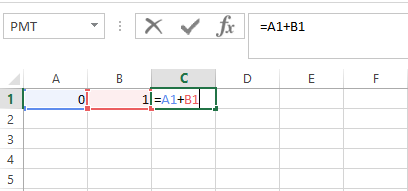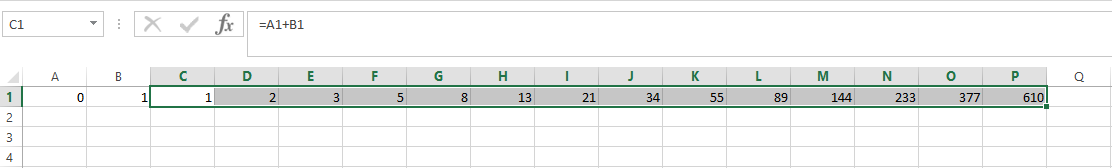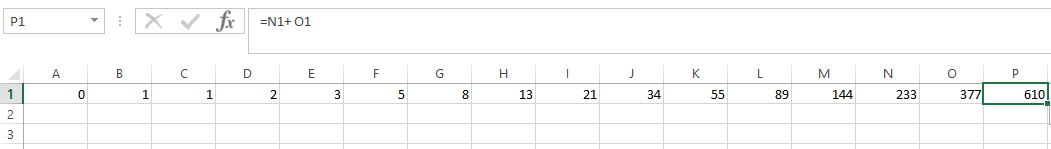#### How to Create Fibonacci Sequence in Excel

This is Excel tutorial on how to make Fibonacci numbers form a sequence. After two starting numbers, the next number in the sequence is the sum of the two preceding numbers.

The Fibonacci ten numbers in the Fibonacci sequence are: 0, 1, 2, 3, 5, 8, 13, 21, 44, 65.

## Preparing Fibonacci sequence formula

Here are the steps to creating a Fibonacci sequence in Excel:

Click on cell A1 and type the number “0” in it.

Click on cell B1 and type the number “1” in it.

Click on cell C1 and type “=A1+B1” in it## How to Create Fibonacci Sequence?

To copy the formula to the adjacent cells, place the mouse pointer on the bottom right corner of cell C1. You will see a black plus sign. Drag the plus sign to cell P1. You will see the number “612” in cell P1.(optional) To continue the Fibonacci series, continue dragging to the right.This will generate the Fibonacci sequence automatically.

## Sum formula to create Fibonacci Sequence

To create a Fibonacci sequence in Excel, you can use a combination of the SUM function and a formula to generate the sequence. Here’s how to create a Fibonacci sequence in Excel:

• In cell A1, enter “Number”.
• In cell B1, enter “Fibonacci Sequence”.

Enter the initial values of the sequence:

• In cell A2, enter “1”.
• In cell A3, enter “2”.
• In cell B2, enter “1”.
• In cell B3, enter “1”.

Enter the formula:

• In cell B4, enter the formula: =B2+B3.
• Copy the formula down the column for as many terms as you want in the sequence.

Autofill the number column:

• In cell A4, enter the formula: =A3+1.
• Select cell A4 and the cells below it.
• Click and drag the autofill handle (bottom right corner of the cell) down the column to fill in the numbers.

With this simple formula, you can create a Fibonacci sequence in Excel that calculates and displays the terms of the sequence based on the previous two terms.

## SEQUENCE Function

The SEQUENCE function allows you to generate a sequence of numbers in a column or row. You can set the starting value, the number of rows or columns, and the step size. For Fibonacci sequences, you can start with “0” and use a step size of “1” to create the sequence.

Example: =SEQUENCE(10,1,0,1)

This formula generates a column of 10 Fibonacci numbers.

## FILL Function

The FILL function is another way to create sequences in Excel. You can specify the starting value, the step size, and the number of values you want to generate.

Example: =FILL(0,1,10)

This formula generates the same 10 Fibonacci numbers as the previous example.

## LET Function

The LET function allows you to define variables and use them in your calculations. This can be handy for generating Fibonacci sequences, especially if you want to include additional logic or calculations.

Example: =LET(n, 10, seq, SEQUENCE(n, 1, 0, 1), seq)

This formula generates a column of 10 Fibonacci numbers using the LET function.

See also  How to create a dynamic pivot table and refresh automatically

## Custom Functions for Fibonacci Sequences

For more complex Fibonacci sequence requirements or if you want to create custom sequences, you can consider creating your own custom Excel functions using VBA (Visual Basic for Applications). Custom functions offer greater flexibility and can incorporate specific logic or calculations.

```Function FibonacciSequence(n As Integer) As Variant
Dim i As Integer
Dim Seq() As Double
ReDim Seq(1 To n)

Seq(1) = 0
Seq(2) = 1

For i = 3 To n
Seq(i) = Seq(i - 1) + Seq(i - 2)
Next i

FibonacciSequence = Seq
End Function```

This VBA custom function, named FibonacciSequence, takes an integer n as input and returns an array of n Fibonacci numbers.